# A mix of Roman, Greek, and single letter index entries

I am typing my thesis and have a mix of regular words, mathematics using greek symbols, and the occasional one letter word (such as the programming language C), and I noticed that my system for ensuring these are sorted correctly has fallen short.

# An example of the problem

Notice where the `\$chi^2\$` appears in the index: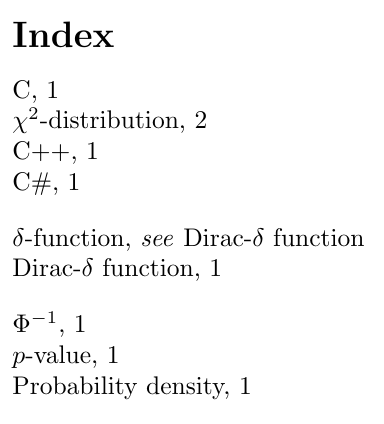whereas what I am after is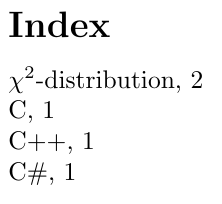# My current system

1. If a regular roman word then just use index as intended such as `index{Something}`, including for single letter words such as `index{C}` or `index{R}`.
2. If there is a roman letter which I want to come first but in math mode then I use a single underscore, such as `index{p_value@{\$p\$-value}}` or `index{t_distribution@{\$t\$-distribution}}`. The underscore ensures these appear first in their sort field.
3. If there is a leading greek mathematical letter then I want this to appear before the roman entries, so I use a double underscore such as `index{d_delta_function@{\$delta\$-function}}`.

Where possible I try and use a leading textual entry and so would use `Dirac-\$delta\$ function` over `\$delta\$-function`. However, I would use the greek symbols in preference to the greek letter spelt out, and so would use `\$beta\$-radiation` rather than `beta-radiation`.

# One workaround

I notice that if I write `Cindex{C@{C}}` rather than `Cindex{C}` then the sorting appears to be better, but I haven’t tested this fully and it smells like a bad practice. I have also in the past accidentally duplicated many index entries by having a trailing space such as `index{Something }` which is a pain to debug, so am apprehensive about encouraging that I add one or two trailing spaces to every index.

How can I get the desired sorting, and how should I revise my indexing system for future documents?

# MWE

``````documentclass{article}
usepackage{makeidx}
makeindex
begin{document}
index{d_delta_function@{\$ delta \$-function}|see {Dirac-\$ delta \$ function}}

The Cindex{C}
%Cindex{C@{C}} % <-- This gives better sorting.
language is great, and C++index{C++} is okay, as too is \$ C \$#index{C_sharp@{C#}}, but if you want to compute a \$ p \$-valueindex{p_value@{\$ p \$-value}} for a Gaussian random variable with inverse cumulative distribution function \$ Phi^{-1} \$index{p__phi_inverse@{\$ Phi^{-1} \$}} then you may encounter difficulties with the probability densityindex{Probability density} if it tends to a Dirac-\$ delta \$index{Dirac_delta_function@{Dirac-\$ delta \$ function}} function.

clearpage
This becomes worse if you want to handle a \$ chi^2 \$-distributionindex{C@{\$ chi^2 \$-distribution}}

printindex
end{document}
``````

# Related questions

Get this bounty!!!

## #StackBounty: #np-hardness #sorting #minimization when inserting some elements to an array which contains bubbles, what is minimum move…

### Bounty: 50

Given:

(1) An array $$A[0$$ : $$m-1]$$ with the length of $$m$$, e.g., $$m=7$$, then $$A$$=$$[1, nil, nil, nil, 4, 4, nil]$$

(2) $$A[0$$ : $$m-1]$$ contains $$n$$($$) elements and $$m-n$$ bubbles, e.g., $$n=3$$, then $$A$$=$$nil$$, $$A$$=$$nil$$, $$A$$=$$nil$$, $$A$$=$$nil$$;

(3) There may be duplicate elements between the $$n$$ elements, e.g., $$A==A$$;

Question:

When inserting $$k$$ ($$k+nleq m$$) elements into the array $$A[0$$ : $$m-1]$$, we MUST sort the array $$A[0$$ : $$m-1]$$ in ascending order, the only operations we can take are:

(1) moving an existing element to a bubble

(2) inserting a new element to a bubble

how to calculate the moving scheme with the minimum number of moves (since the $$k$$ inserting operations are unavoidable) or is it NP-hard?.

e.g., $$m=7$$, $$A[0$$ : $$m-1]$$=$$[1, nil, nil, nil, 4, 4, nil]$$;
$$k=3$$, the $$k$$ elements to be inserted are $$4$$, $$5$$, and $$5$$.

One possible moving scheme:

step 1: move the element in $$A$$ to $$A$$. After that, $$A[0:6]$$ is $$[1, nil, 4, nil, 4, nil, nil]$$;

step 2: insert $$4$$, $$5$$, and $$5$$ into $$A$$, $$A$$, and $$A$$. After that, $$A[0:6]$$ is $$[1, 4, 4, nil, 4, 5, 5]$$;

Get this bounty!!!

## #StackBounty: #table-of-contents #sorting Sort the list of figures by caption

### Bounty: 100

I would like to sort the list of figures by caption alphabetically (instead of the order of appearance).

Something like

``````List of figures

4 Aaaa      3
1 Bbbbb     1
8 Ddd dd    10
``````

Is there a way?

Get this bounty!!!

## #StackBounty: #unity #2d #rpg #sorting Issue with Sorting Layers with an NPC and Tilemap

### Bounty: 50

I have a weird thing happening, that I can’t quite explain. I have two scenes, my starting town, and an Arcanist’s shop. When I go into the Arcanist’s Shop, the Arcanist NPC stays on the same sorting layer (background layer 0) as my checkered tiles, and the table is background layer 1. When I start the game, the NPC will switch between being “behind” the table, or on top of it whenever I reenter the Arcanist’s Shop scene. It seems to switch between background layer 0 and 1 with no cause.

I’ve double checked my sorting layers, they’re all where they should be. I’ve checked the camera’s culling mask, it’s fine. I’ve checked to see if there are any weird components on anything in my shop scene, and there aren’t

See the image attached, this is the same instance of the game, just different times when I enter the Arcanist’s Shop.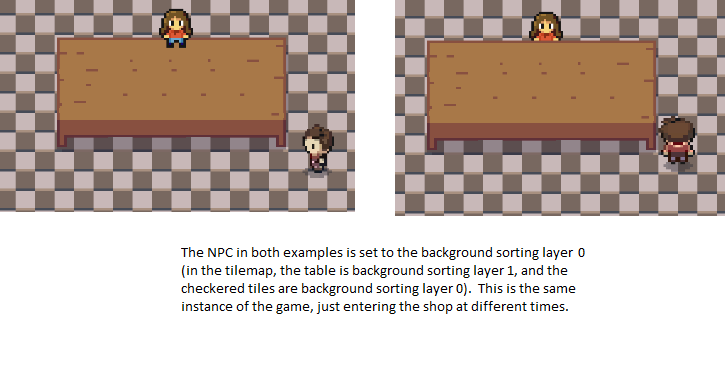Screenshot of my tilemap setup: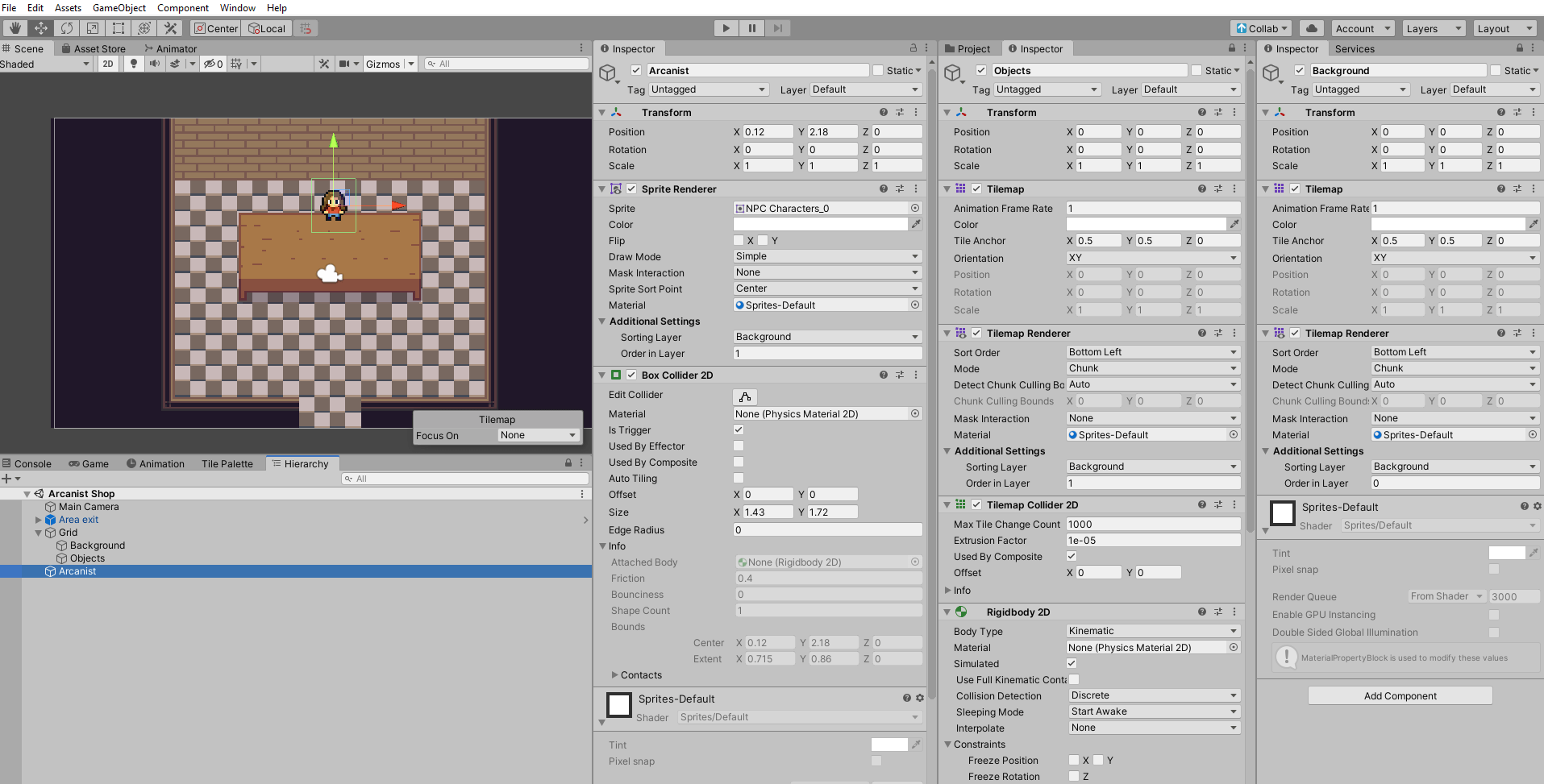Get this bounty!!!

### Bounty: 100

Apologies if this question feels like a solution verification, but this question was asked in my graduate admission test and there’s a lot riding on this:

What is the worst case time complexity of inserting $$n$$ elements into an empty linked list, if the linked list needs to be maintained in sorted order?

In my opinion, the answer should be $$O(n^2)$$ because in every insertion, we will have to insert the element in the right place and it is possible that every element has to be inserted at the last place, giving me a time complexity of $$1 + 2 + … (n-1) + n = O(n^2)$$

However, the solution that I have says that we can first sort the elements in $$O(n log n)$$ and then, we can insert them one by one in $$O(n)$$, giving us an overall complexity of $$O(n log n)$$.

From the given wording of the question, which solution is more apt? In my opinion, since the question mentions “linked list needs to be maintained in sorted order”, I am inclined to say that we cannot sort the elements beforehand and then insert them in the sorted order.

Get this bounty!!!

## #StackBounty: #magento2 #collection #product-collection #sorting Magento2: I want to show featured product on top of the category and s…

### Bounty: 50

I have yes/no type product attribute.

If this featured attribute is yes for any products, those product will show top(first featured product showing in category page then rest of the products are showing) in the category page including search result as well.

What is the best way to achieve this so it will work perfectly if catalog flat is Enabled.

Get this bounty!!!

## #StackBounty: #algorithms #sorting #weighted-graphs #database-theory What are the common practices to weight tags relations?

### Bounty: 50

I am working on a webapp (fullstack JS) where the user create documents and attach tags to them. They also select a list of tags they are interested in and attach them to their profile.

I am not a math guy, but I did some NLP as hobbyist and learnt about latent semantic indexation: as I understand it, you create a table where you store each couple of words you parsed, and then add weight to each of these couples of words when both are found next to each other.

I was thinking of doing the same thing with tags: when 2 tags appear on the same document or profile, I increase the weight of their couple. That would allow me to get a ranking of the “closest” tags of a given one.

Then I remembered that I came across web graphs, where websites were represented in a 2D space (x and y coordinates) and placed depending on their links using an algorithm called force vector.

While I do know how I would implement my first idea, I am not sure about the second one. How do I spread the tag coordinates when created? Do they all have an `x:0, y:0` at the start?

Since I assume this is a common case of data sorting, I wondered what would be the common/best practices recommended by people of the field.

Is there documents, articles, libraries (npm?) or wikipedia pages you could point me out to help me understand what can or should ideally be done? Is my first option a good one by default?

Also, please let me know in comments if I should add or remove a tag to this question or edit its title: I’m not even sure of how to categorize it.

Get this bounty!!!

## #StackBounty: #magento2 #database #magento2.3 #sql #sorting Magento 2: Multiple Product Type Not Sorting Due To Amasty Extension

### Bounty: 50

I’m facing a problem due to Amasty Extension. I have a category which has 1 `simple product` and some `configurable products`, but when I try to sort them, simple product always comes first although configurable product did sort as I need but simple product always comes first here are some screenshots:

You can see I sort `simple product` on 5th position but it always comes first, so I try to debug and echo the `Magento_Catalog/templates/product/list.phtml`

``````\$_productCollection = \$block->getLoadedProductCollection();
echo get_class(\$_productCollection);
echo "<br>".\$_productCollection->getSelect()->__toString();
``````

And I got this query with class that goes to `Amasty`

``````AmastyShopbyModelResourceModelFulltextCollectionInterceptor

SELECT
`e`.*,
`cat_index`.`position` AS `cat_index_position`,
`price_index`.`price`,
`price_index`.`tax_class_id`,
`price_index`.`final_price`,
IF(price_index.tier_price IS NOT NULL,
LEAST(price_index.min_price,
price_index.tier_price),
price_index.min_price) AS `minimal_price`,
`price_index`.`min_price`,
`price_index`.`max_price`,
`price_index`.`tier_price`,
`stock_status_index`.`stock_status` AS `is_salable`
FROM
`catalog_product_entity` AS `e`
INNER JOIN
`catalog_category_product_index_store1` AS `cat_index` ON cat_index.product_id = e.entity_id
AND cat_index.store_id = 1
AND cat_index.visibility IN (2 , 4)
AND cat_index.category_id = 59
INNER JOIN
`catalog_product_index_price` AS `price_index` ON price_index.entity_id = e.entity_id
AND price_index.website_id = '1'
AND price_index.customer_group_id = '2'
LEFT JOIN
`cataloginventory_stock_status` AS `stock_status_index` ON e.entity_id = stock_status_index.product_id
AND stock_status_index.website_id = 0
AND stock_status_index.stock_id = 1
WHERE
(e.entity_id IN ((SELECT
`cat`.`product_id`
FROM
`catalog_category_product_index_store1` AS `cat`
WHERE
(cat.category_id IN ('206')))))
ORDER BY `cat_index`.`position` ASC ,  `cat_index`.`position` ASC
LIMIT 18
``````

I found out that WHERE is the problem here:

``````WHERE
(e.entity_id IN ((SELECT
`cat`.`product_id`
FROM
`catalog_category_product_index_store1` AS `cat`
WHERE
(cat.category_id IN ('206')))))
``````

So I replace this WHERE with INNER JOIN

``````INNER JOIN
`catalog_category_product_index_store1` AS `cat` ON cat.product_id = e.entity_id
AND cat.store_id = 1
AND cat.visibility IN (2 , 4)
AND cat.category_id IN ('206')
``````

after that I got product sorting like this: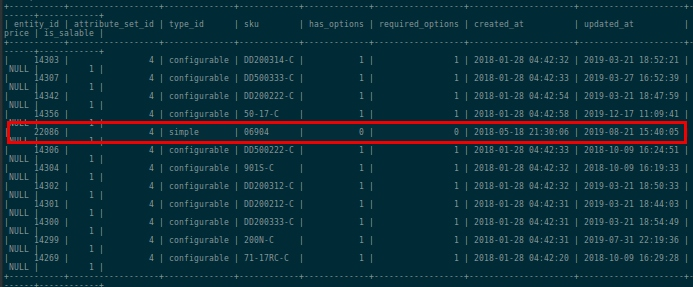You can see with the INNER JOIN I got all the product at the exact position as I want it to be. So I need to apply it in Magneto and I found that class I mention before `AmastyShopbyModelResourceModelFulltextCollection.php`, I start debugging and found that this function made the WHERE query:

``````public function addIndexCategoriesFilter(array \$categoriesFilter)
{
foreach (\$categoriesFilter as \$conditionType => \$values) {
\$categorySelect = \$this->getConnection()->select()->from(
['cat' => \$this->getTable('catalog_category_product_index')],
'cat.product_id'
)->where(\$this->getConnection()->prepareSqlCondition('cat.category_id', ['in' => \$values]));
\$selectCondition = [
\$this->mapConditionType(\$conditionType) => \$categorySelect
];
\$whereCondition = \$this->getConnection()->prepareSqlCondition('e.entity_id', \$selectCondition);
\$this->getSelect()->where(\$whereCondition);
\$this->requestBuilder->bind(Category::ATTRIBUTE_CODE, \$values);
}
return \$this;
}
``````

I try to replace WHERE with INNER JOIN but I don’t know how to do that, please help me out with this.

Thank You…

Get this bounty!!!

## #StackBounty: #bibtex #natbib #sorting In bibliographystyle{alpha}, can I sort references by the first author's last name instead …

### Bounty: 50

I’m creating a bibliography using `bibliographystyle{alpha}`. Consider the following three references.

[Gab72] Peter Gabriel…

[GJ09] Paul G. Goerss and John F. Jardine…

[GR92] Peter Gabriel and Andrei V. Roiter…

They are automatically being displayed in my LaTeX file in the ordering above. This is alphabetical order by the marker: [Gab72] < [GJ09] < [GR92]. Is it possible to instead have the ordering based on alphabetical ordering of the first author’s last name? This would instead give the following ordering:

[Gab72] Peter Gabriel…

[GR92] Peter Gabriel and Andrei V. Roiter…

[GJ09] Paul G. Goerss and John F. Jardine…

I would prefer this latter ordering because then Peter Gabriel’s references are adjacent.

Get this bounty!!!

## #StackBounty: #bibtex #natbib #sorting In bibliographystyle{alpha}, can I sort references by the first author's last name instead …

### Bounty: 50

I’m creating a bibliography using `bibliographystyle{alpha}`. Consider the following three references.

[Gab72] Peter Gabriel…

[GJ09] Paul G. Goerss and John F. Jardine…

[GR92] Peter Gabriel and Andrei V. Roiter…

They are automatically being displayed in my LaTeX file in the ordering above. This is alphabetical order by the marker: [Gab72] < [GJ09] < [GR92]. Is it possible to instead have the ordering based on alphabetical ordering of the first author’s last name? This would instead give the following ordering:

[Gab72] Peter Gabriel…

[GR92] Peter Gabriel and Andrei V. Roiter…

[GJ09] Paul G. Goerss and John F. Jardine…

I would prefer this latter ordering because then Peter Gabriel’s references are adjacent.

Get this bounty!!!